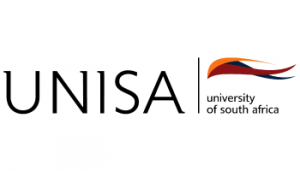relevant institution directly for course information.

# Register Here

FOR ALL THE LATEST OFFERS
AND SPECIALS NOW

Register
What are you looking for?# COURSE: BSC IN MATHEMATICAL AND STATISTICAL SC...N/A
###### Duration:
3 Years Full Time / Full Time / Part Time
This degree is aimed at students interested in using their mathematical and statistics skills to solve problems in manufacturing, banking, business management and management consultancy. Apart from strengthening their knowledge of advanced mathematics and statistics, students are introduced to business, financial modelling, financial risk modelling, production modelling, forecasting and strategic decision-making models.

Duration: 3 years part time

#### Compulsory Subjects

##### First year subjects:

• Descriptive statistics

• Probability and probability distributions

• Statistical inference

• Data analysis

• Pre-calculus Mathematics

• Calculus

• Linear algebra

• Introduction to the business world

• Modelling in a financial environment

• End-user Computing

• Introduction to Business Information Systems

• Comprehension skills for science

##### Second year subjects:

• Distribution theory

• Applied statistics

• Forecasting

• Linear algebra

• Calculus in higher dimensions

• Mathematical programming

• Rational decision making

• Financial modelling

##### Third year subjects:

• Distribution theory

• Inference

• Analysis of variance and regression

Careers: management consultant, risk manager, financial risk manager, production modeller.

Source: www.unisa.ac.za